أخر الاخبار

# How to calculate Land Surface Temperature with Landsat 8 satellite images

## How to calculate Land Surface Temperature with Landsat 8 satellite images

The Land Surface Temperature can be estimated or calculated using the Landsat 8 thermal bands. It simply requires applying a set of equations through a raster image calculator (ArcMap, ArcGIS Pro, QGIS).

The first step is to download a Landsat 8 image from a particular location, unzip it, and check certain information needed (within the metadata) to execute this procedure.

This tutorial shows how to calculate Land Surface Temperature (LST) using the Landsat 8 bands. In particular, band 10 as the thermal band, and bands 4 and 5 to calculate the Normal Difference Vegetation Index (NVDI).

To calculate the LST, use the USGS formulas (more information in the article Algorithm for Automated Mapping of Land Surface Temperature Using LANDSAT 8 Satellite Data), this example simply performs the calculations without going into detail about the fundamentals. The process is synthesized in six steps below:

#### 1.- Calculation of TOA (Top of Atmospheric) spectral radiance.

TOA (L) = ML * Qcal + AL

where:

ML = Band-specific multiplicative rescaling factor from the metadata (RADIANCE_MULT_BAND_x, where x is the band number).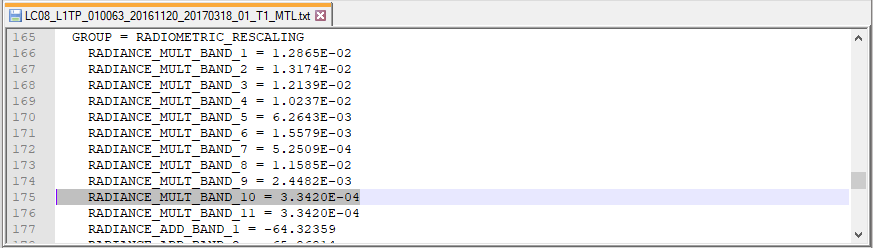Qcal = corresponds to band 10.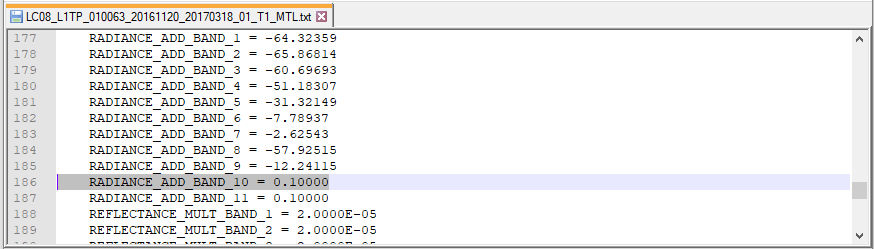TOA = 0.0003342 * “Band 10” + 0.1

Therefore the equation must be solved using the Raster Calculator tool in ArcMap.#### 2.- TOA to Brightness Temperature conversion

BT = (K2 / (ln (K1 / L) + 1)) − 273.15

where:

K1 = Band-specific thermal conversion constant from the metadata (K1_CONSTANT_BAND_x, where x is the thermal band number).
K2 = Band-specific thermal conversion constant from the metadata (K2_CONSTANT_BAND_x, where x is the thermal band number).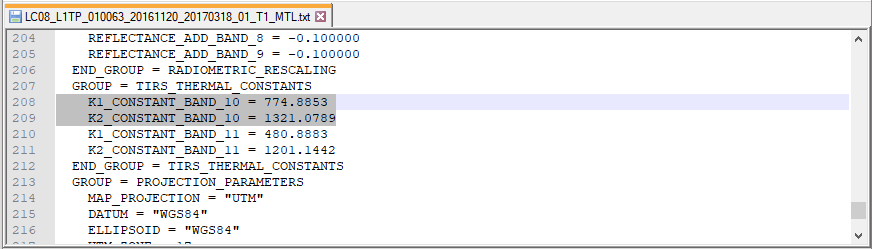L = TOA

Therefore, to obtain the results in Celsius, the radiant temperature is adjusted by adding the absolute zero (approx. -273.15°C).

BT = (1321.0789 / Ln ((774.8853 / “%TOA%”) + 1)) – 273.15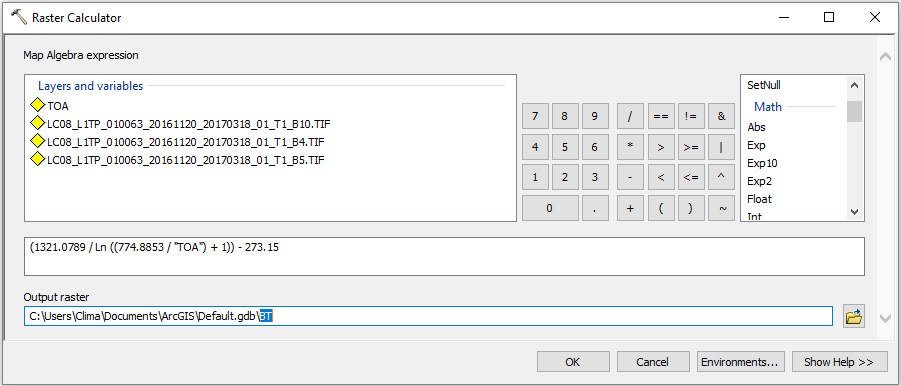#### 3.- Calculate the NDVI

NDVI = (Band 5 – Band 4) / (Band 5 + Band 4)

Note that the calculation of the NDVI is important because, subsequently, the proportion of vegetation (Pv), which is highly related to the NDVI, and emissivity (ε), which is related to the Pv, must be calculated.

NDVI = Float(Band 5 – Band 4) / Float(Band 5 + Band 4)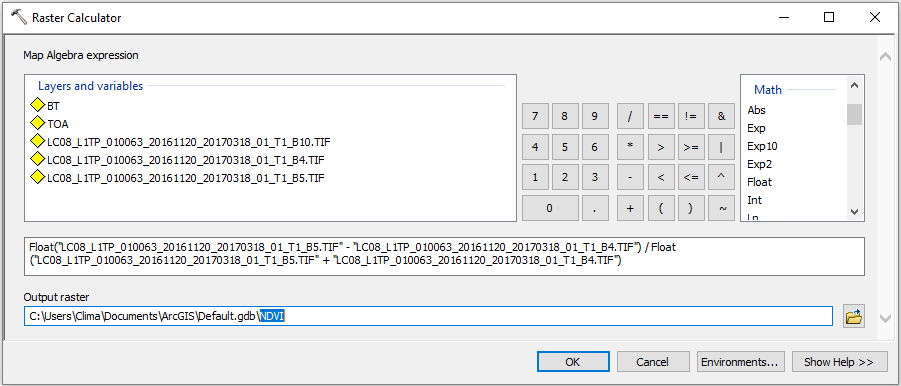#### 4.- Calculate the proportion of vegetation Pv

Pv = Square ((NDVI – NDVImin) / (NDVImax – NDVImin))

Usually the minimum and maximum values of the NDVI image can be displayed directly in the image (both in ArcGIS, QGIS, ENVI, Erdas Imagine), otherwise you must open the properties of the raster to get those values.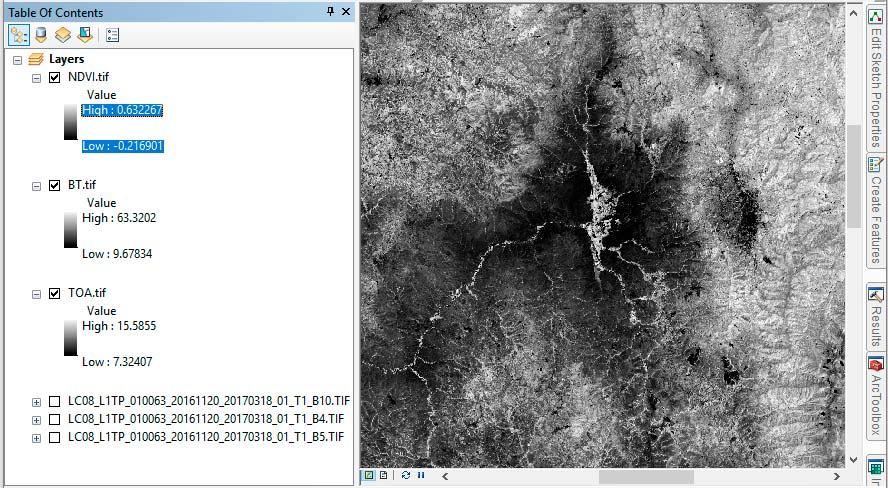Pv = Square((“NDVI” – 0.216901) / (0.632267 – 0.216901))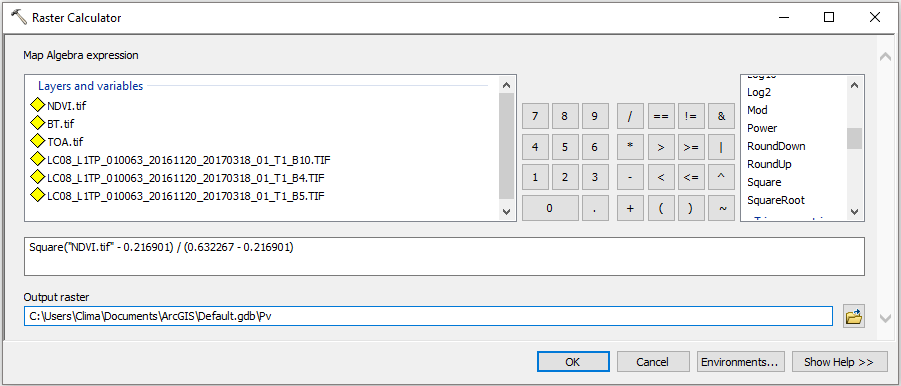#### 5.- Calculate Emissivity ε

ε = 0.004 * Pv + 0.986

Simply apply the formula in the raster calculator, the value of 0.986 corresponds to a correction value of the equation.#### 6.- Calculate the Land Surface Temperature

LST = (BT / (1 + (0.00115 * BT / 1.4388) * Ln(ε)))

Finally apply the LST equation to obtain the surface temperature map.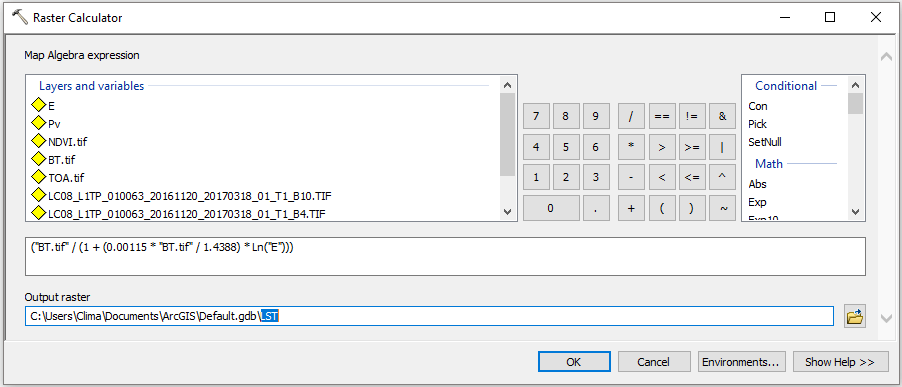As a result of the process developed, there is a map of the Land Surface Temperature, it should be noted that it is not equal to the air temperature.بواسطة : كوكب المنى
تعليقات

حجم الخط
+
16
-
تباعد السطور
+
2
-